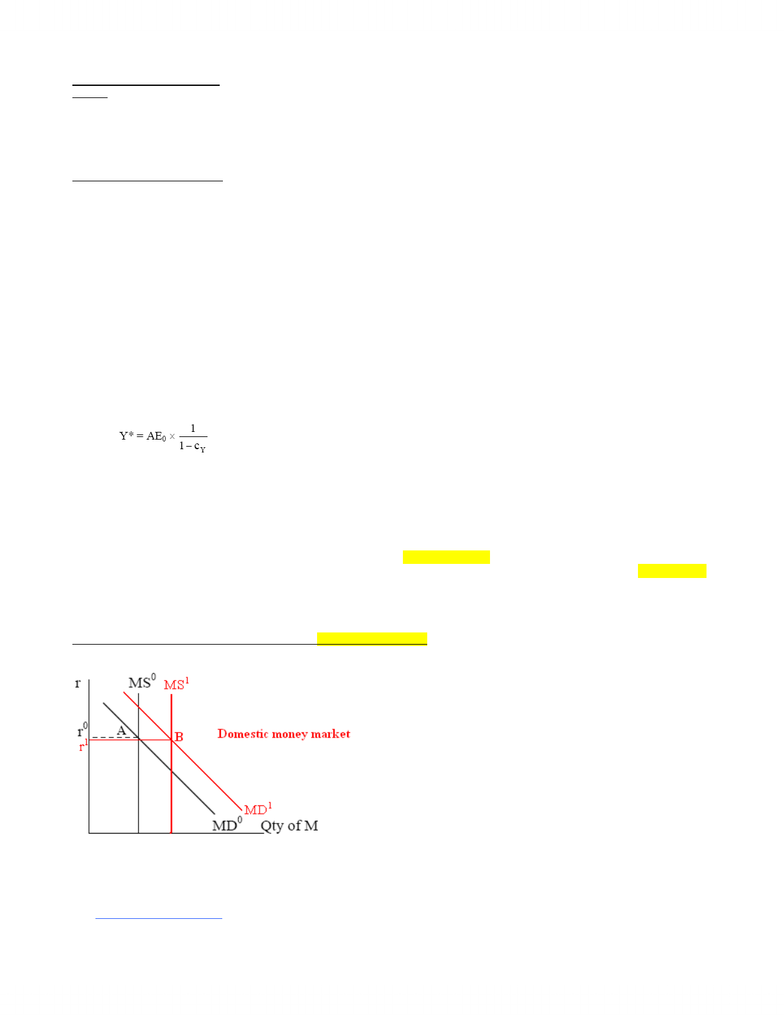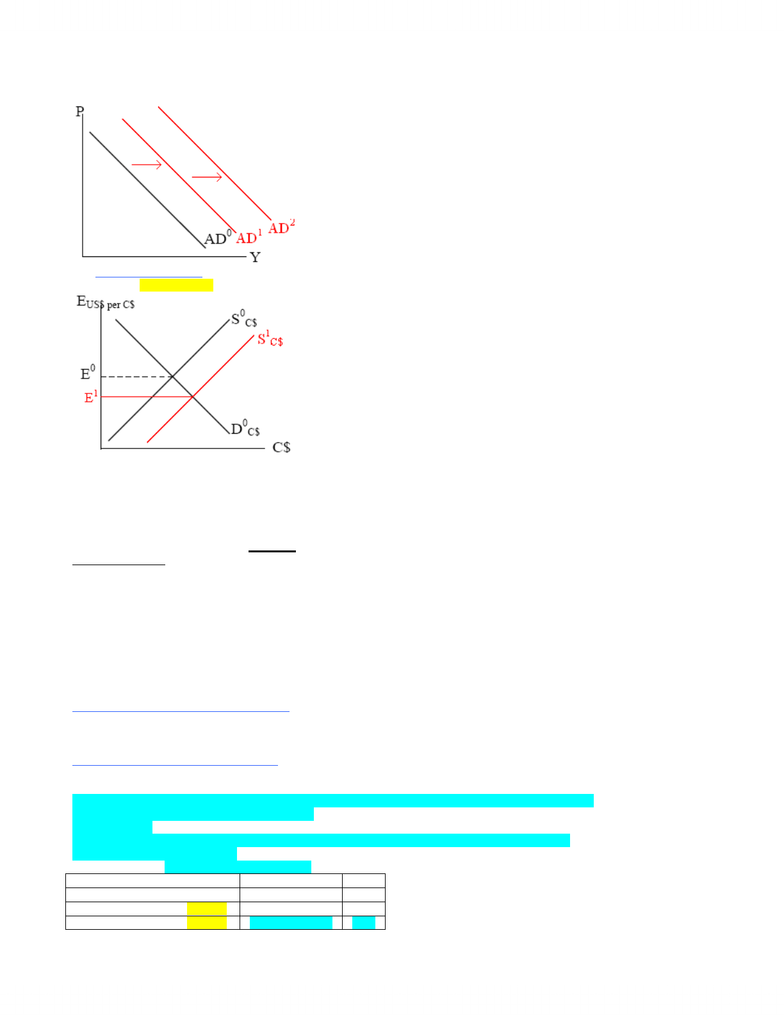# MGEA06H3 Lecture Notes - Fixed Exchange-Rate System, Fiscal Policy, Monetary Policy

79 views5 pagesOPEN ECONOMY (PART 2)
Outline
x A brief review of what we have learned for an open economy.
x Discuss the effectiveness of monetary and fiscal policies under flexible exchange rate.
x Discuss the effectiveness of monetary and fiscal policies under fixed exchange rate.
x Wrap up the course!
Open Economy—A Brief Review
The AE Model in Generic Form—the Full Version
Consumption: C = C0 + c1DI, where DI = Y – T + TR
Investment: I = I0 d (r – r1), where r1 is a constant
Government sector: G
T = T
0 + tr1Y
TR = TR0 – tr1Y
Foreign sector: X = X0 – x1 (E – E1), where E1 is a constant
IM = IM0 + im1Y + im2 (E – E1), where E1 is a constant
x The AE function: AE = C + I + G + X – IM
AE = C0 + c1 [Y – (T0 + tr1Y) + (TR0tr1Y)] + [I0 d (r – r1)] + G + [X0 – x1 (E – E1)] – [IM0 + im1Y + im2 (E – E1)]
AE = C0 + I0 + G + X0 – IM0c1T0 + c1TR0 – d (r – r1) – (x1 + im2) (E – E1) + [c1 (1 – t1 – tr1) – im1] Y
AE = AE0 + cYY,
where AE0 = C0 + I0 + G + X0 – IM0c1T0 + c1TR0 – d (r – r1) – (x1 + im2) (E – E1)
c
Y = c1 (1 – t1 – tr1) – im1
where r1 and E1 is a constant
*** These two terms show how a change in interest rates and exchange rates change the AE function and equilibrium level of output.
x Equilibrium level of output (equating Y = AE):
Observations
x Holding all else constant, a change in E would affect both exports and imports, which then affects AE (via a change in AE0) and Y.
o When C\$ appreciates (E increases), Canadian goods become more expensive Æ X decreases and IM increases Æ AE
decreases Æ Y decreases.
x A change in interest rate has two affects on the economy:
1) It affects the cost of borrowing and then investment.
When r increases, cost of borrowing increases Æ I decreases Æ Y decreases.
2) It also has a significant impact on the exchange rate due to capital movements.
When r increases, Canadian assets become more attractive Æ foreigners buy more Canadian assets Æ capital inflows.
Demand for C\$ increases Æ C\$ appreciates (E increases).
x A change in interest rate will cause the exchange rate to change; therefore, a change in interest rate has a large effect on X and IM.
o r increases Æ C\$ appreciates (E increases) Æ X decreases and IM increases Æ AE decreases Æ Y decreases.
Effectiveness of Monetary and Fiscal Policies under Flexible Exchange Rate
Case 1: Monetary Policy under Flexible Exchange Rate
The central bank runs expansionary monetary policy:
x Suppose the central bank buys bonds, MS increases.
x At r0, people find that they have too much liquidity (excess supply of money), and they will try to get rid of the excess liquidity by
x bond prices increase and interest rate decreases (r decreases)
x r decreases Æ cost of borrowing decreases Æ I increases Æ AE increases Æ Y increases
x The SMALL modification:
x Y increases Æ L (r, Y) increases by a small amount
www.notesolution.com
Unlock document

This preview shows pages 1-2 of the document.
Unlock all 5 pages and 3 million more documents.x overall, r decreases to r1 (pt B in the money market diagram)
x The BIG modification: When r (interest rate) decreases, Canadian assets become less attractive Æ Canadians buy more foreign
assets Æ capital outflows.
x SC\$ shifts to the right to S1C\$ in the foreign exchange market
x C\$ depreciates to E1 (E decreases) Æ X increases, IM decreases Æ AE increases further Æ AD shifts further to the right to AD2
x Y increases further due to all these increases
x The first increase in AD is due to an increase in MS
x The second increase in AD is due to an increase in NX
x Conclusion: Monetary policy is effective (it can be used to affect Y) under flexible exchange rate. Because the effect of an
expansionary monetary policy is reinforced by an increase in NX.
Numerical Example
C = 20 + 7/8 DI
T = (2/7) Y – 20
TR = 220 – (1/7) Y
I = 100 – 500 (r – 0.05)
G = 250
X = 120 – 1000 (E – 0.85); E = # of US\$ per C\$
IM = 1/8 Y + 750 (E – 0.85)
x If r = 0.05 (i.e., 5%) and E = 0.85:
o AE function: AE = 700 + 3/8 Y
o Equilibrium: Y* = 1120
Week 8—No Change in E When MS Changes:
x Now, suppose the central bank increases the money supply, and the interest rate falls to 3% (r = 0.03) and E remains at 0.85:
o AE function: AE = 710 + 3/8 Y
o Equilibrium: Y* = 1136 (Y increases by 16)
Week 12—Change in E When MS Changes:
x Now, suppose the central bank increases the money supply and the interest rate falls to 3% (r = 0.03) and C\$ depreciates to 0.83 (E
decreases to 0.83):
AE = (230 + ½ Y) + [100 – 500 (0.03 – 0.05)] + 250 + [120 – 1000 (0.83 – 0.85)][1/8 Y + 750 (0.83 – 0.85)]
AE = 230 + ½ Y + 110 + 250 + 140 – 1/8 Y – (–15)
AE = 745 + 3/8Y
AE0 increased because I increased by 10, X increased by 20, and IM decreased by 15 (from the initial case)
Equilibrium: Y = 745 + 3/8Y
Y* = 1192 (Y* increases by 72)
AE function Y*
Initial case, r = 0.05, E = 0.85 AE = 700 + 3/8 Y 1120
MS increases, r = 0.03, E = 0.85 AE = 710 + 3/8 Y 1136
MS increases, r = 0.03, E = 0.83 AE = 745 + 3/8 Y 1192
www.notesolution.com
Unlock document

This preview shows pages 1-2 of the document.
Unlock all 5 pages and 3 million more documents.

# Get access

\$10 USD/m
Billed \$120 USD annually
Homework Help
Class Notes
Textbook Notes